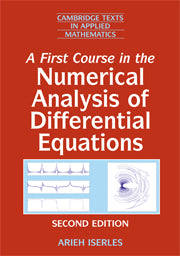# A First Course in the Numerical Analysis of Differential Equations by Iserles, Arieh

• R 644.00
Unit price per
Tax included.

ISBN: 9780521734905

Cambridge University Press | 01 May 2015

Paperback | 480 pages

Numerical analysis presents different faces to the world. For mathematicians it is a bona fide mathematical theory with an applicable flavour. For scientists and engineers it is a practical, applied subject, part of the standard repertoire of modelling techniques. For computer scientists it is a theory on the interplay of computer architecture and algorithms for real-number calculations. The tension between these standpoints is the driving force of this book, which presents a rigorous account of the fundamentals of numerical analysis of both ordinary and partial differential equations. The exposition maintains a balance between theoretical, algorithmic and applied aspects. This second edition has been extensively updated, and includes new chapters on emerging subject areas: geometric numerical integration, spectral methods and conjugate gradients. Other topics covered include multistep and Runge-Kutta methods; finite difference and finite elements techniques for the Poisson equation; and a variety of algorithms to solve large, sparse algebraic systems.